0
2471

# Direction Sense Questions For MAH MBA CET

Download MAH MBA CET Direction Sense Questions and Answers PDF covering the important questions. Most expected Directions test reasoning questions with explanations for MAH MBA CET / MMS CET exam.

Question 1: Ashok started walking towards South. After walking 50 meters he took a right turn and walked 30 meters. He then took a right turn and walked 100 meters. He again took a right turn and walked 30 meters and stopped. How far and in which direction was he from the starting point?

a) 50 meters South

b) 150 meters North

c) 180 meters East

d) 50 meters North

e) None of these

Question 2: Town D is towards East of town F. Town B is towards North of town D. Town H is towards South of town B. Towards which direction is town H from town F?

a) East

b) South-East

c) North-East

e) None of these

Question 3: A person rode his bicycle Northwards, then turned left and rode one km and again turned left and rode 2km. He found himself exactly one km West of his starting point. How far did he ride Northwards initially?

a) 1 km

b) 2km

c) 3km

d) 4km

e) 5km

InstructionsDirections : Study the following information carefully and answer the given questions.

Question 4: A school bus driver starts from the school, drives 2 km towards North, takes a left turn and drives for 5 km. He then takes a left turn and drives for 8 km before taking a left turn again and driving for further 5 km. The driver finally takes a left turn and drives 1 km before stopping. How far and towards which direction should the driver drive to reach the school again?

a) 3km towards North

b) 7 km towards East

c) 6 km towards South

d) 6 km towards West

e) 5 km towards North

Question 5: Point R is 10 metres north of point A. Point K is exactly in the middle of the points R and A. Point N is 7 metres east of point A. Point M is 7 metres east of point K. Point S is 6 metres north of point M. What is the distance between points S and N?

a) 13 metres

b) 16 metres

c) 11 metres

d) 12 metres

e) None of these

Instructions

Study the given information and answer the given questions.
Point A is 11 m North of point B.
Point C is 11 m East of point B
Point D is 6 m North of point C
Point E is 7 m West of point D
Point F is 8 m North of point E
Point G is 4 m West of point F

Question 6: What is the shortest distance between point F from point A ?

a) 43m

b) 4 m

c) 3 m

d) 7 m

e) 5 m

Question 7: How far and in which direction is point G from point A ?

a) 3 m North

b) 5 m North

c) 4 m North

d) 4 m South

e) 3 m South

Question 8: Mohan walked 30 meters towards South, took a left turn and walked 15 meters. He then took a right turn and walked 20 meters. He again took a right turn and walked 15 meters.How far is he from the starting point ?

a) 95 meters

b) 50 meters

c) 70 meters

d) Cannot be determined

e) None of these

Question 9: Ashok started walking towards South. After walking 50 meters he took a right turn and walked 30 meters. He then took a right turn and walked 100 meters. He again took a right turn and walked 30 meters and stopped. How far and in which direction was he from the starting point ?

a) 50 meters South

b) 150 meters North

c) 180 meters East

d) 50 meters North

e) None of these

Question 10: Town D is towards East of town F. Town B is towards North of town D. Town H is towards South of town B. Towards which direction is town H from town F?

a) East

b) South-East

c) North-East

e) None of these

Question 11: Q walked 20 metres towards West, took a left turn and walked 20 metres.He then took a right turn and walked 20 metres and again took a right turn and walked 20 metres.How far is Q now from the starting point ?

a) 40 meters

b) 50 metres

c) 80 metres

e) None of these

Question 12: Gautham starts from point A and rides towards north for 4 kms to reach B, then he takes left turn and travel for 8 kms to reach point C and then he takes another left turn and travel for 12 kms to reach point D. Finally he takes another left turn and travel for another 8kms to reach point E. What is the current position of Gautham with respect to the point A?

a) 8 kms towards east

b) 12 kms towards south

c) 12 kms towards west

d) 8 kms towards south

e) Cannot be determined

Question 13: Ganesh starts from point A and travels towards the east for 36 km to reach point B, at B he takes a right turn and travels for 77 km to reach point C.
What is the distance between the points A and C?

a) 75

b) 89

c) 86

d) 85

e) none of these

Question 14: Karan starts from point A and travels towards north for 5 km. He then takes a right turn and travels for 7 km. He then takes a left turn and travels another 7 km. Finally he takes another left turn and travels for 2 km arriving at a point B.
What is the distance between point A and B?

a) 13 km

b) 12.33 km

c) 12 km

d) 10 km

e) None of these

Question 15: Prajwal starts from point P and travels towards the east for 5km and then he turns right to travel for another 5km and then he turns to his left and reaches point B after travelling 7km.
What is the distance between P and B?

a) 12 km

b) 13 km

c) 9 km

d) 11 km

e) None of these

Question 16: Anup starts from point A and travels towards north for 8 km and then he turns to his left and travels for 15 km to reach point B.
What is the distance between A and B and the direction to which Anup is finally facing?

a) 17 km, West.

b) 23 km, East.

c) 19 km, West.

d) 21 km, East.

e) None of these

Instructions

Read the given information carefully and answer the given questions. B is 25 m south of A. C is 10 m east of B. D is 30 m north of C. E is 7 m east of D. X is 18 m south of E. M is 12 m south of X. C is 7 m west of M.

Question 17: B is in which direction from Point D ?

a) South

b) South­West

c) North­East

d) South­East

e) North

Question 18: What is the distance between B and M?

a) 17 m

b) 15 m

c) 21 m

d) 19 m

e) 13 m

Instructions

Study the given information carefully to answer the given questions.
Point E is 16 m to the south of Point C. Point F is 4 m to the west of Point E. Point H is 5 m to the south of Point F. Point J is 12 m to the east of Point H. Point Y is 27 m to the east of Point F. A person walks 15 m from Point Y towards west, reaches Point Z, takes a left turn and reaches Point J.

Question 19: If a person walks 20m towards north from Point Z, takes a left turn and walks 8 m, how far will be from Point C ?

a) 2 m

b) 6 m

c) 3 m

d) 4 m

e) 5 m

Question 20: In which direction is Point Z with respect to Point H?

a) Northwest

b) Northeast

c) Southwest

d) East

e) South east

Ashok walked 50m towards South. Then he took right and walked 30m=> he is travelling towards East. Now, he is 50m South and 30m East from his starting position.

Then he took right and travelled 100m => Now he is walking towards North and is 50m North and 30m East from his starting position.

Finally, he took right again and travelled 30m => He is walking towards West and is 50m North from his starting position.

The below are the different possible positions of the cities:

B                     OR                   B                  OR                     B

F          H/D                                   F      D                                           H

H                           F               D

Hence H can be South-East of F or East of F or North-East.

According to the question, way of riding of the person is as follow.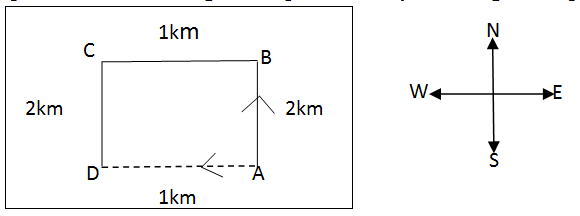As per the question, D is 1km West of A, therefore AB=DC=2km.

The driver drove 5 km west, 5 km east, 8 km south and 2+1 = 3 km north. So, he has to travel a distance of 8 – 3 = 5 km north to reach his starting point.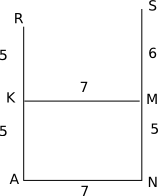The required distance = 11 m

$AF^{2} = 4^{2} + 3^{2}$

AF = 5

According to the above diagram, shortest distance between F and A will be 5 m. Hence, answer will be E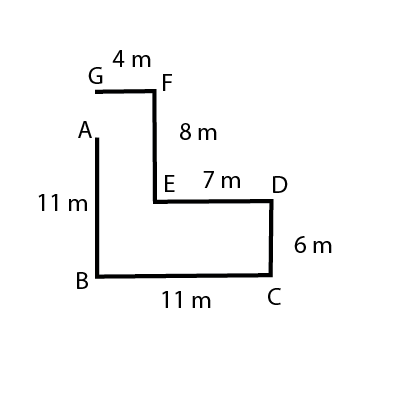As we can see in the above diagram that distance between G and A will be 8-(11-6) = 3 m

The movement of Mohan is as shown in the diagram below. So, he is 30 + 20 = 50 metres from the original spot.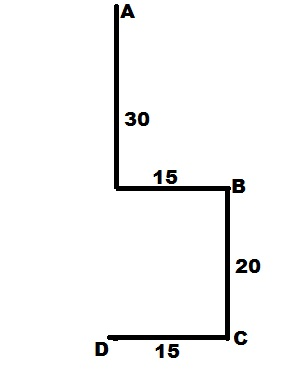The movement of Ashok is as shown below. So, he is North of the original spot by 100 – 50 = 50 metres.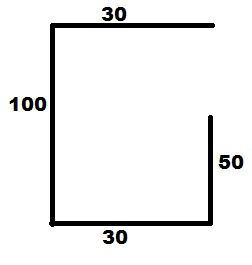The position of H can be on either side of D as shown in the diagram below. Unless that is specified, we can’t say if H is to the North East or South East of F.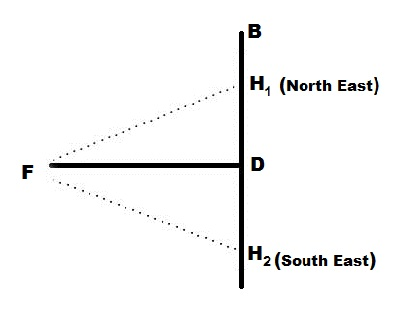The movement of Q is as shown in the diagram below. So, he is at a distance of 40 metres from the original point.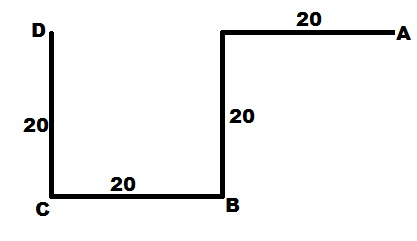Based on the given information, the path traveled by lakshman could be derived as below.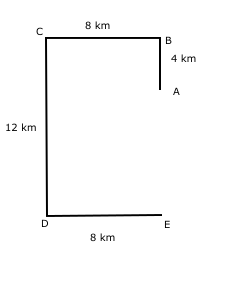The final point E is 8 km towards south of point A.

So the correct option to choose is D – 8 km towards south.

Based on the given information, the path travelled by Ravi could be as below.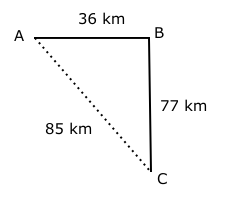Distance between A and C = $\sqrt{77^{2} + 36^{2}}$

So the correct option to choose is C – 85

Based on the given information, the path travelled by karan could be derived as below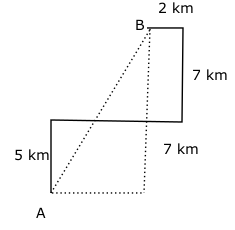Therefore the distance between A and B = $\sqrt{12^{2} + 5^{2}}$ = 13

So the correct option to choose is A – 13 km.

Based on the given information, the path travelled by Prajwal could be as below.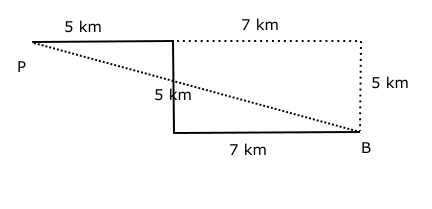The distance between P and B = $\sqrt{5^{2} + 12^{2}}$ = 13 km.

So the correct option to choose is B-13 km.

Based on the given information, the path travelled by Anup could be as below.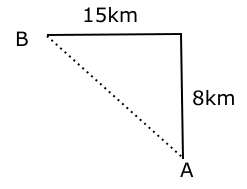Distance between A and B = $\sqrt{8^2 + 15^2}$ = 17 km.

Anup faces west at point B.

So the correct option to choose is A.

Based on the above conditions, the points when plotted on a graph will be :

where the numbers in the brackets is the distance between two points.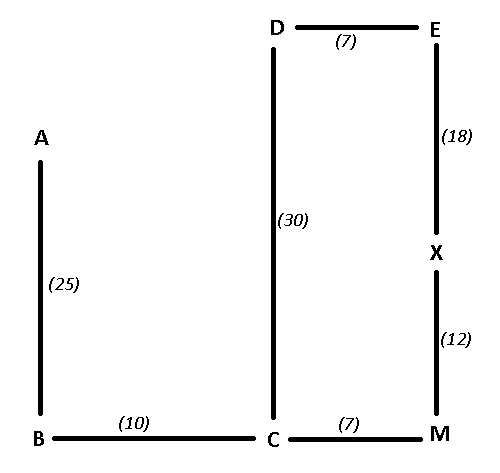B is in south-west direction from point D.

Thus, Ans – (B)

Based on the above conditions, the points when plotted on a graph will be :

where the numbers in the brackets is the distance between two points.As seen from above, distance between B and M = 10+7 = 17

Thus, Ans – (A)

Y is 27m to the east of point F. When one walks 15m to the west from point Y, he reaches Z.

=> Z is 12m to the east of point F and 8m to the east of point E.

Distance between F and H is 5m which is also equal to the distance between Z and J.

Based on the above conditions, these points when plotted on a graph will be :

The numbers in the brackets is the distance between any two points.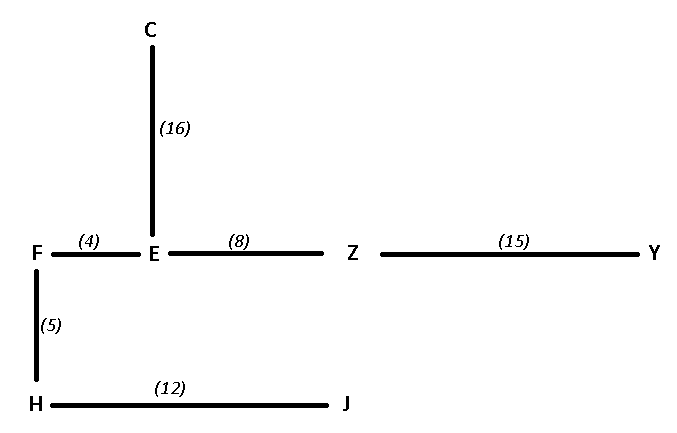If a person walks 20 m north from point Z and then take left turn and walk 8 m .

He will be = 20-16 = 4m to the north of point C.

Thus, Ans – (D)

Y is 27m to the east of point F. When one walks 15m to the west from point Y, he reaches Z.

=> Z is 12m to the east of point F and 8m to the east of point E.

Distance between F and H is 5m which is also equal to the distance between Z and J.

Based on the above conditions, these points when plotted on a graph will be :

The numbers in the brackets is the distance between any two points.Clearly, Z is to the north-east of H.

Thus, Ans – (B)